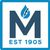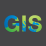# Graph Labels NOT Showing Correctly

615
3
09-10-2013 08:21 AMOccasional Contributor III
Having issues with the titles (X-Axis) and (Y-Axis) in my graphs not working properly. I have it set in a Python script to make it "KWH_USAGE" but when the graph is created - it shows "kwh_usage"... Why is it only showing everything as lower case?

Also - I want to include the data from a specific field in a feature class to show in the title. So if I have 14884512 in a field called "TWACs_Number" - I want the title to include that. How would I write that?

Thank you
Tags (3)
1 Solution

Accepted SolutionsbyEsri Esteemed Contributor
Chris,

I believe I was assisting your before.  I think the title of the axis's being lowercase is a bug.  I was able to reproduce this same behavior.  I would recommend following up with Tech Support.

As for the title, you can use the 'getValue' method to retrieve the value of your field.  Ex:

`import arcpy from arcpy import env env.overwriteOutput = 1 env.workspace = r"C:\temp\data.gdb"  table = "Meters"  fields = ["METER_NUMBER"]  list = []  rows = arcpy.SearchCursor(table) for row in rows:     list.append(row.METER_NUMBER)  del row, rows  #remove duplicates from list list = dict.fromkeys(list) list = list.keys()  for n in list:     arcpy.TableSelect_analysis(table, r"in_memory\table_sel", "METER_NUMBER = " + str(n))          #get TWACs_Number value     for row in arcpy.SearchCursor(r"in_memory\table_sel"):         TWACs_Number = row.getValue("TWACs_Number")          out_graph_name = n     out_graph_pdf = r"C:\Temp" + "\\" + str(n) + ".pdf"     input_template = r"C:\Temp\KW Demand.grf"     input_data = r"in_memory\table_sel"      # Create the graph     graph = arcpy.Graph()      # Add a vertical bar series to the graph     graph.addSeriesBarVertical(input_data, "KW_DEMAND")      # Specify the title of the left axis     graph.graphAxis.title = "KW Demand"      # Specify the title of the bottom axis     graph.graphAxis.title = "Meter Number"      # Specify the title of the Graph     graph.graphPropsGeneral.title = TWACs_Number      # Output a graph, which is created in-memory     arcpy.MakeGraph_management(input_template, graph, out_graph_name)      # Save the graph as a PDF     arcpy.SaveGraph_management(out_graph_name, out_graph_pdf, "MAINTAIN_ASPECT_RATIO", 600, 375)`
3 RepliesbyEsri Esteemed Contributor
Chris,

I believe I was assisting your before.  I think the title of the axis's being lowercase is a bug.  I was able to reproduce this same behavior.  I would recommend following up with Tech Support.

As for the title, you can use the 'getValue' method to retrieve the value of your field.  Ex:

`import arcpy from arcpy import env env.overwriteOutput = 1 env.workspace = r"C:\temp\data.gdb"  table = "Meters"  fields = ["METER_NUMBER"]  list = []  rows = arcpy.SearchCursor(table) for row in rows:     list.append(row.METER_NUMBER)  del row, rows  #remove duplicates from list list = dict.fromkeys(list) list = list.keys()  for n in list:     arcpy.TableSelect_analysis(table, r"in_memory\table_sel", "METER_NUMBER = " + str(n))          #get TWACs_Number value     for row in arcpy.SearchCursor(r"in_memory\table_sel"):         TWACs_Number = row.getValue("TWACs_Number")          out_graph_name = n     out_graph_pdf = r"C:\Temp" + "\\" + str(n) + ".pdf"     input_template = r"C:\Temp\KW Demand.grf"     input_data = r"in_memory\table_sel"      # Create the graph     graph = arcpy.Graph()      # Add a vertical bar series to the graph     graph.addSeriesBarVertical(input_data, "KW_DEMAND")      # Specify the title of the left axis     graph.graphAxis.title = "KW Demand"      # Specify the title of the bottom axis     graph.graphAxis.title = "Meter Number"      # Specify the title of the Graph     graph.graphPropsGeneral.title = TWACs_Number      # Output a graph, which is created in-memory     arcpy.MakeGraph_management(input_template, graph, out_graph_name)      # Save the graph as a PDF     arcpy.SaveGraph_management(out_graph_name, out_graph_pdf, "MAINTAIN_ASPECT_RATIO", 600, 375)`Occasional Contributor III
Jake-

Yes - huge help on one of my previous posts to get the Python script to create the graph. Nice to know that there is a bug in the tool/script. I have been trying multiple different things for the past few days to get it to work. I will send a note to Tech Support and see what can be done. Thank you for the help.

Chris

Chris,

I believe I was assisting your before.  I think the title of the axis's being lowercase is a bug.  I was able to reproduce this same behavior.  I would recommend following up with Tech Support.

As for the title, you can use the 'getValue' method to retrieve the value of your field.  Ex:

```import arcpy
from arcpy import env
env.overwriteOutput = 1
env.workspace = r"C:\temp\data.gdb"

table = "Meters"

fields = ["METER_NUMBER"]

list = []

rows = arcpy.SearchCursor(table)
for row in rows:
list.append(row.METER_NUMBER)

del row, rows

#remove duplicates from list
list = dict.fromkeys(list)
list = list.keys()

for n in list:
arcpy.TableSelect_analysis(table, r"in_memory\table_sel", "METER_NUMBER = " + str(n))

#get TWACs_Number value
for row in arcpy.SearchCursor(r"in_memory\table_sel"):
TWACs_Number = row.getValue("TWACs_Number")

out_graph_name = n
out_graph_pdf = r"C:\Temp" + "\\" + str(n) + ".pdf"
input_template = r"C:\Temp\KW Demand.grf"
input_data = r"in_memory\table_sel"

# Create the graph
graph = arcpy.Graph()

# Add a vertical bar series to the graph

# Specify the title of the left axis
graph.graphAxis.title = "KW Demand"

# Specify the title of the bottom axis
graph.graphAxis.title = "Meter Number"

# Specify the title of the Graph
graph.graphPropsGeneral.title = TWACs_Number

# Output a graph, which is created in-memory
arcpy.MakeGraph_management(input_template, graph, out_graph_name)

# Save the graph as a PDF
arcpy.SaveGraph_management(out_graph_name, out_graph_pdf, "MAINTAIN_ASPECT_RATIO", 600, 375)```New Contributor

Just ran into the same problem and am wondering if there is a workaround?

Thanks.

Fred